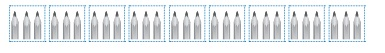Home | | Maths 3rd Std | Number operations in daily life situations

# Number operations in daily life situations

Example numerical problems with solutions : Number operations in daily life situations

Number operations in daily life situationsExample 1

If the cost of one eraser is 4, what will be the cost of 2 erasers?4 + 4 = 8

2 × 4 = 8

The cost of two erasers = 2 × 4 = 8

Example 2

If there are 6 eggs in a box, how many eggs will be there in 5 boxes.Number of Boxes = 5

Number of Eggs = 6

Total Number of Eggs = 5 × 6 = 30

Exercise

1. If there are 4 toys in a box. How many toys will be there in 9 boxes?Number of Boxes = 9

Number of toys in each box = 4

Total Number of toys = 9 × 4 = 36

2.  If there are 3 colour pencils in a packet. How many colour pencils will be there in 9 packets?Number of packets = 9

Number of colour pencils in each packets = 3

Total Number of colour pencils = 9 × 3 = 27

3. Write the multiplication fact for the following products24

3 × 8 ; 12 × 2 ; 2 × 12 ; 1 × 24 ; 24 × 1

45

15 × 3 ; 3 × 15 ; 45 × 1 ; 1 × 45

36

3 × 12 ; 18 × 2 ; 2 × 18 ; 36 × 1 ; 1 × 36

16

2 × 8 ; 4 × 4 ; 8 × 2 ; 16 × 1 ; 1 × 16Tags : Numbers | Term 2 Chapter 1 | 3rd Maths , 3rd Maths : Term 2 Unit 1 : Numbers
Study Material, Lecturing Notes, Assignment, Reference, Wiki description explanation, brief detail
3rd Maths : Term 2 Unit 1 : Numbers : Number operations in daily life situations | Numbers | Term 2 Chapter 1 | 3rd Maths